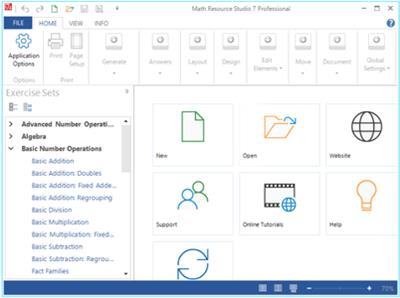; charset=UTF-8" /> Math Resource Studio Professional v7.0.158 | CSKsiteSep 06

# Math Resource Studio Professional v7.0.158

Math Resource Studio Professional v7.0.158Math Resource Studio Professional v7.0.158 | 53 MB | Language: English

[spoiler]
Generate Printable Math Worksheets and Activities. Generate individual or class sets of math worksheets, workbooks, or tests quickly and effortlessly. Provide students with the precise skills development and math practice they need as part of a differentiated numeracy program.

Motivate Students
Math Resource Studio is the ideal math worksheet creation tool because it makes it easy for teachers to provide that motivating match between learning activity and learning need.
Target Learning Needs
Math Resource Studio makes it easy to create differentiated math worksheets to target the learning needs of all of your students. Generate worksheets with the exact practice required to advance skills to the next level.
Simple, Powerful, Unique
Producing math practice worksheets with Math Resource Studio is as simple as selecting the types of questions you want, setting a few options, and clicking the generate button to create a completely original math worksheet.
Math activities
A total of more than 120 unique math worksheet activities in all. And with the many optional settings for each activity, there are literally hundreds of activity configurations available.
– basic number operations
– number concepts
– algebra
– geometry
– fractions
– numeration
– number lines
– time
– consumer math
– math puzzles
– ratio and percent
– measurement
– tables and drills
– graphing
– coordinates
A basic math worksheet in seconds
It takes just a few clicks of the mouse to create an original math worksheet with basic questions for addition, subtraction, multiplication, and division.
– Select from over 120 math question generators.
– Generate new questions with a single click.
– Shuffle questions with just a click.
– Add a customizable title group.
Math puzzles
Improve math skills with high-interest math puzzle activities.
– Includes magic squares, number patterns, addition boxes, sudokus, and more.
– Make the puzzles easier or more difficult using the many design options.
Tables and drills
Provide essential practice for the basic math facts.
– Includes addition, subtraction, multiplication, division, and counting.
– Makes great timed warm-up activities.
– Control the level of difficulty.
– Target specific math facts.
Word problems
Easily create word problems for basic number operations. Simply click to add tags for numbers, names, and objects. Tags are then replaced with automatically generated values.
– Tags for numbers, names, and objects are replaced with generated values.
– Create your own lists of objects and names.
– Set the range for the generated numbers.
– Includes basic number operations, fractions, consumer math, percent, and more.
Basic number operations
Create worksheets with up to 19 activities for practice in basic addition, subtraction, multiplication, and division.
– Complete control over the range of values used for the generated numbers.
– Select vertical or horizontal question layout.
– Automatically generate up to 100 questions for each exercise set.
– Optionally provide answer banks for self correction.
Number Concepts
Worksheet practice for a variety of number concepts including exponents, roots, scientific notation, and more.
– Practice solving simple expressions with exponents from -9 to 9 including 0.
– Convert numerals from Arabic to Roman or Roman to Arabic.
– Practice with scientific notation up to 10 quadrillion.
– Solve for any or for all of mean, median, mode, and range.
Requirements:Windows 10, 8, 7
[/spoiler]

Homepage: https://www.schoolhousetech.com